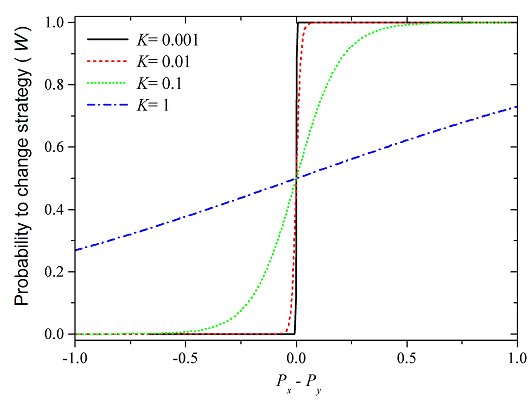Figure 2: K is free parameter and means a temperature, which defines a level of irrational decisions within PD. If K is small, i.e. practically zero, the level of uncertainty is also zero. If K is large, this implies a strongly uncertain environment where all information are always lost. The intermediate region of K is the most interesting. When the level of uncertainty is low, which is indicated with small K = 0.01 (the red line), the probability of adopting chosen strategy is lower than by higher level of uncertainty with K = 0.1 (the green line).